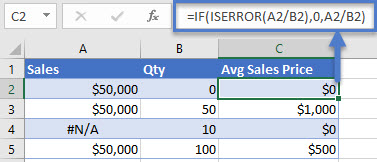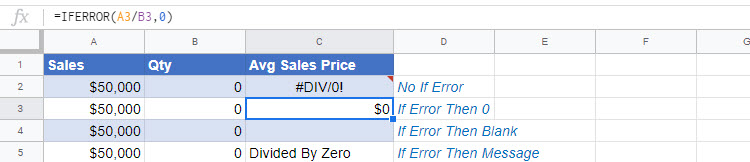### Reddit Example

<<I will provide intro content here. including listing out the IFERROR syntax>>

## What Is the IFERROR Function?

The IFERROR function falls under the category of Logical Functions in Microsoft Excel, which includes ISNA, ISERROR and ISERR. All of these functions help detect and handle formula errors.

IFERROR allows you to perform a calculation. If the calculation does not result in an error, then the calculation result is displayed. If the calculation does result in an error then another calculation is performed (or a static value like 0, blank, or some text is outputted).

When would you use the IFERROR Function?

• When dividing numbers to avoid errors caused by dividing by 0
• When performing lookups to prevent errors if the value isn’t found.
• When you want to perform another calculation if the first results in an error (ex. Lookup a value in a 2nd table if it’s not found in the first table)

Un-handled formula errors can cause errors within your workbook, but visible errors also make your spreadsheet less visibly appealing.

### If Error Then 0

Let’s look at a basic example. Below you are dividing two numbers.  If you attempt to divide by zero you will receive an error: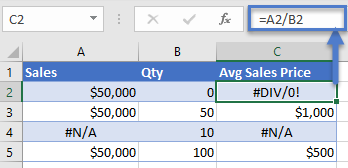Instead, insert the calculation within the IFERROR function and if you divide by zero a 0 is outputted instead of an error:

`=IFERROR(A2/B2,0)`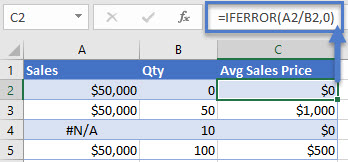### If Error Then Blank

Instead of setting errors to 0, you can set them to ‘blank’ with double quotations (“”):

`=IFERROR(A2/B2,"")`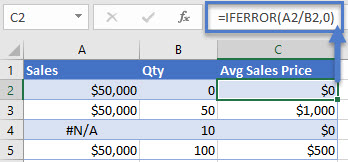### If Error Then Do Something Else

The IFERROR Function can also be used to perform a 2nd calculation if the 1st calculation results in an error. We will use VLOOKUPs in the example below:

<<code>>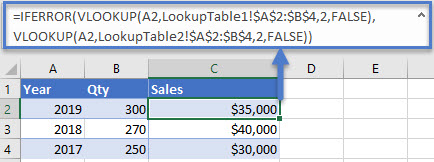## More IFERROR Formula Examples

### Nested IFERROR – VLOOKUP Multiple Sheets

You can nest an IFERROR inside another IFERROR to perform 3 separate calculations. Here we will use two IFERRORs to perform VLOOKUPs on 3 separate worksheets: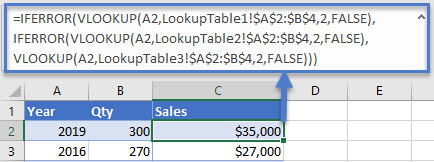### Index / Match & XLOOKUP

Of course, IFERROR will also work with Index / Match and XLOOKUP formulas as well.

#### IFERROR XLOOKUP

XLOOKUP function is an advanced version of VLOOKUP function.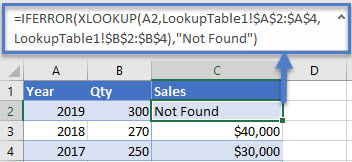#### IFERROR INDEX / MATCH

You can also lookup values by using INDEX and MATCH functions in Excel.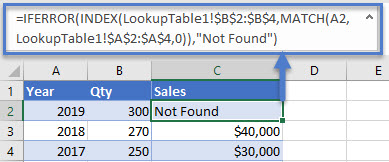If you don’t know how XLOOKUP function or MATCH/INDEX function works, then click on this link to find a detailed explanation.

### IFERROR in Arrays

Array formulas in Excel are used to perform several calculations through a single formula. Let’s suppose there are three columns of Year, Sales, and Avg Price. You can find out the total quantity with the following formula in the E column.

`{=SUM(\$B\$2:\$B\$4/\$C\$2:\$C\$4)}`

The formula performs well until the divisor range gets an empty cell or zeroes. As a result, you get to see the #DIV/0!error again.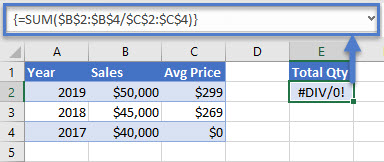This time, you can use the IFERROR function like this:

`{=SUM(IFERROR(\$B\$2:\$B\$4/\$C\$2:\$C\$4,0))}`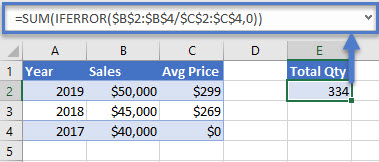Notice that the IFERROR function must be nested inside the SUM Function, otherwise the IFERROR will apply to the sum total and not each individual item in the array.

### IFNA vs. IFERROR

The IFNA Function works exactly the same as the IFERROR Function except the IFNA function will only catch #N/A errors. This is useful when working with lookup functions: Only “lookup value not found errors are handled”, normal formula errors can still appear alerting you to problems with your calculation.

<<example>>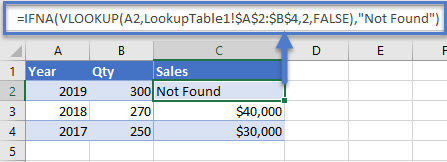### If ISERROR

If you are still using Microsoft Excel 2003 or an older version, then you can substitute IFERROR with a combination of IF and ISERROR. Here is a brief example.

`=IF(ISERROR(A2/B2),0,A2/B2)`